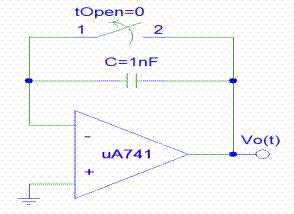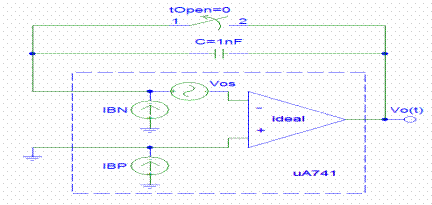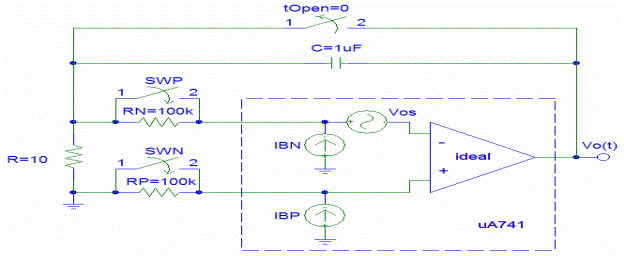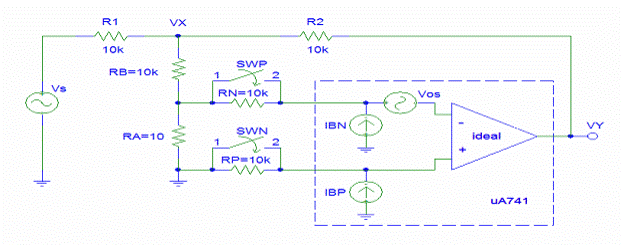Experiment No: 1.

Title: a) To plot DC transfer characteristic of differential amplifier.

b) To study effect of offset voltage on output of preamplifier using general purpose Op

Amp and precision Op Amp.

AIM : 1.To plot DC transfer characteristic of differential amplifier.

2. To study effect of offset voltage on output of preamplifier using general purpose Op Amp and precision Op Amp.

APPARATUS REQUIRED:

Bread board, dual power supply, CRO, IC 741, Tackle box, Jumper wires.

THEORY:

Op Amp Bias, Offset, and Slew Rate:

In this experiment, we will determine the input bias currents and offset voltage of an op amp using two different measurement techniques. In the first method, we will observe the rate at which the small bias current charges the feedback capacitor. In the second method, weâ€™ll use a circuit that amplifies the small voltage at the input of the op amp 1,000 times so that the full DC transfer characteristic of the op amp can be traced in the scopeâ€™s XY mode. Finally, weâ€™ll measure a dynamic op amp limitation known as the maximum slew rate and determine the full power bandwidth.

Saturation Caused by Bias Currents:

The first experiment will demonstrate that even very small bias currents quickly drive the op amp into saturation if no DC path is provided between the input pins and ground.

Capacitor Charging Method:

Weâ€™ll now expand the circuit of Fig. 1A to enable us to determine both bias currents and the offset voltage.

DC Transfer Characteristic Method:

The effect of bias currents and offset voltage can be visualized by tracing the DC transfer characteristics of the op amp. However, due to the very high DC gain of an op amp, the input voltage of the op amp in the linear regime is very low (too low to display on the scope) and the input source instability of even 0.1mV is sufficient to cause large swings of the output voltage. Both problems can be minimized by the use of the circuit shown in Fig. 3A. The negative feedback provided by the inverter configuration of 1 R and 2 R stabilizes the output by having it track the negative of the source voltage s V . In addition, voltageX V is 1,001 times larger than the voltage across the resistor R , which makes the small op amp input voltage detectable with a scope.

Slew Rate:

We know that the linear effect due to the limited bandwidth of an op amp limits the rise time of the output due to a step input function. However, op amps also exhibit non linear distortion due to the limited charging currents available inside the op amp device. Consequently, the rate of change of the output cannot exceed a certain fixed value, known as the slew rate in volts per second.

Circuit Diagram:Fig. 1A Bias currents start charging the capacitor as soon as the switch is opened.Fig. 1B Equivalent circuit of the setup shown in Fig. 1A.Fig. 2A Bias and offset determination via the capacitor charging method.Fig. 2B Equivalent circuit of the setup shown in Fig. 2A.Fig. 3A Bias and offset determination from the DC transfer characteristic.Fig. 3B Equivalent circuit of the setup shown in Fig. 3A.

Procedure:

A. Saturation Caused by Bias Currents

1. Build a circuit shown in Fig. 1A

2. Connect the op ampâ€™s output to the scope (5V/div, 200ms/div)

3. Observe the waveform when the switch is opened:

i. start with the switch closed

ii. Open the switch

iii. Hit Run/Stop button to freeze scope display

iv. Zoom in to more accurately determine the slope

4. From the scope, determine the charging rate of the capacitor: Î” V / Î”t

5. Calculate the bias current of the inverting pin (see Fig. 1B): BN o I = âˆ’C Î”V Î”t

B. Capacitor Charging Method

1. Build the circuit shown in Fig. 2A.

2. Repeat the steps of part A with both switches SWP and SWN closed.

3. From the charging rate determine the offset voltage (see Fig. 2B): VOS = RC Î”Vo Î”t

4. Repeat the steps 3 and 4 of part A with switch SWN open and SWP closed.

5. From the charging rate calculate the bias current BN I (see Fig. 2B):

OS N BN o V âˆ’ R I = RC Î”V Î”t

6. Repeat the steps 3 and 4 of part A with switch SWP open and SWN closed.

7. From the charging rate calculate the bias current PN I (see Fig. 2B):

OS P BN o V + R I = RC Î”V Î”t

8. Calculate the offset current: OS BP BN I = I âˆ’ I

C. DC Transfer Characteristic Method

1. Build the circuit shown in Fig. 3A use Â±5V to power up the op amp.

2. Connect node VX to the Channel 1 of the scope, and VY to Channel 2.

3. Use XY mode and inverted display for Channel 1.

4. For excitation, use 10Vpp sinusoid at 0.1Hz (period equals 10 sec.)

5. With switches SWP and SWN closed, record the transfer characteristic.

6. Determine the offset voltage from: 1001 OS X V = V, where VX is the voltage at the transition point from negative to positive saturation.

7. With switch SWN open and switch SWP closed, record the transfer characteristic.

Design with Op Amps

8. Determine the input bias current from: VOS âˆ’ RN IBN = VX 1001, where VX is the voltage at the transition point from negative to positive saturation.

9. With switch SWN closed and switch SWP open, record the transfer characteristic.

10. Determine the input bias current from: 1001 OS P BP X V + R I =V, where VX is the voltage at the transition point from negative to positive saturation.

11. Calculate the offset current: OS BP BN I = I âˆ’ I

OBSERVATION TABLE:

A.

 Sr. No. Vout VOS = RC Î”Vo Î”t V âˆ’ R I = RC Î”V Î”t Voltage on CRO

B.

 Sr. No. Vout V + R I = RC Î”V Î”t VOS = RC Î”Vo Î”t V o âˆ’ R I = RC Î”V Î”t OFFSET Voltage

C.

 Sr. No. X V =V Offset Voltage V + RI = RC Î”V Î”t V OS = RC Î”Vo Î”t V OS âˆ’ RN IBN =V X 1001 offset current: I = I âˆ’ I X V + R I =V
###### Conclusion:

------------------------------------------------------------------------------------------------------------------------------------------------------------------------------------------------------------------------------------------------------------------------------------------------------------------------------------------------------------------------------------------------------------------------------------

FaceBook
Likes
Additonal Information